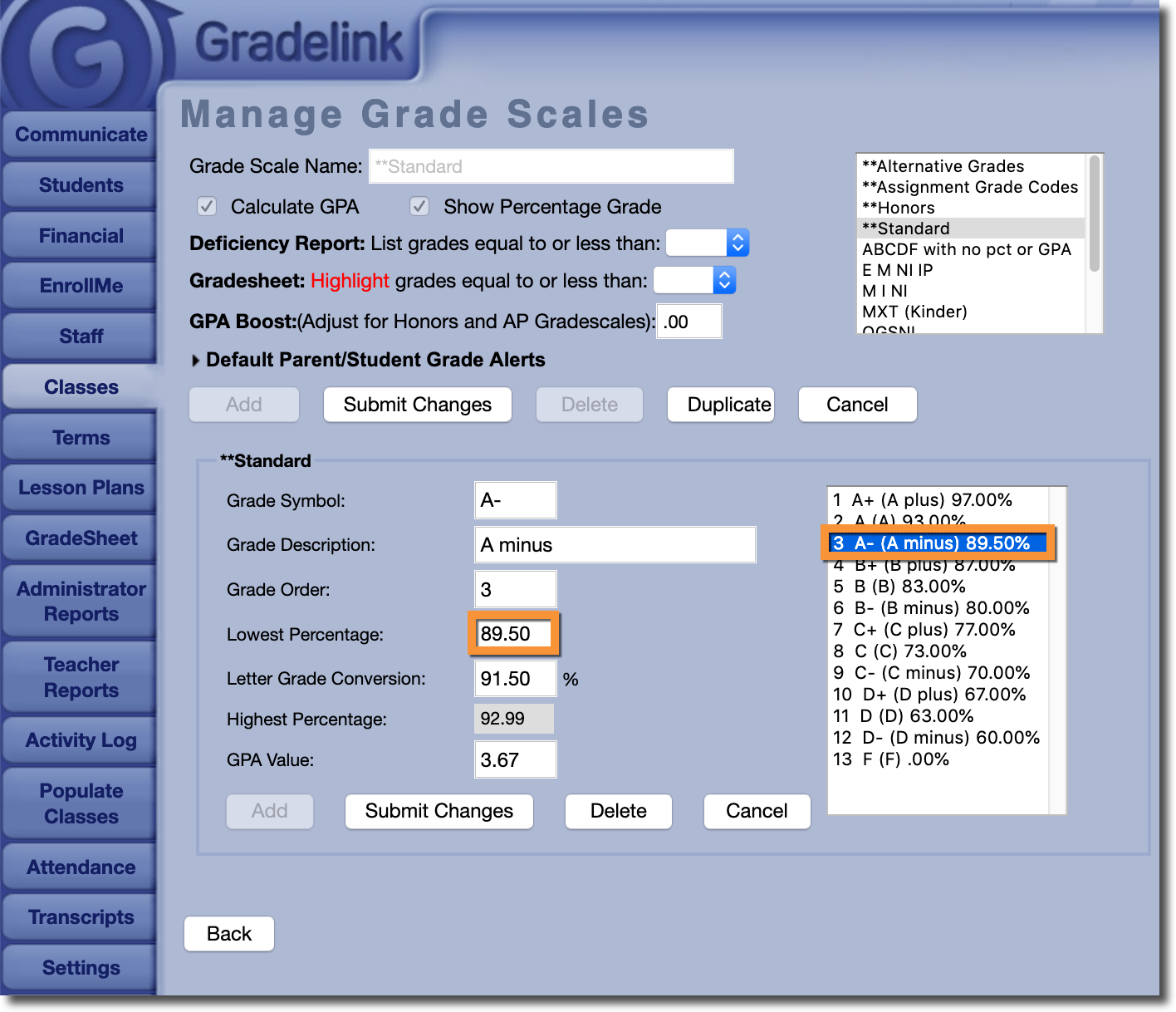Possible Scenario:

Solution:

Therefore, Gradelink does not automatically round up grades that are on the cusp of another higher or lower grade. The only exception is "Double Rounding" on Report Cards; you can read more about "Double Rounding" here.

If you would like to enable rounding on assignments and overall grades (as in, you would like grades that are within a certain margin from a higher or lower grade to be 'bumped' to that grade), you can do so by modifying the Highest and Lowest Percentages on the grade scale assigned to a class.

For example, let's assume that on a particular grade scale, an "A-" has a Lowest Percentage of 90.0%, and a "B+" has a Highest Percentage of 89.99%. If you would like a grade such as an 89.5% that would normally qualify as a "B+" to 'round up' to an "A-", you would need to adjust the Lowest Percentage for an "A-" to be 89.5% (the Highest Percentage for each grade is calculated automatically, so you don't have to worry about adjusting it).

With this change in place, grades such as an 89.5% would now 'round up' to an "A-".